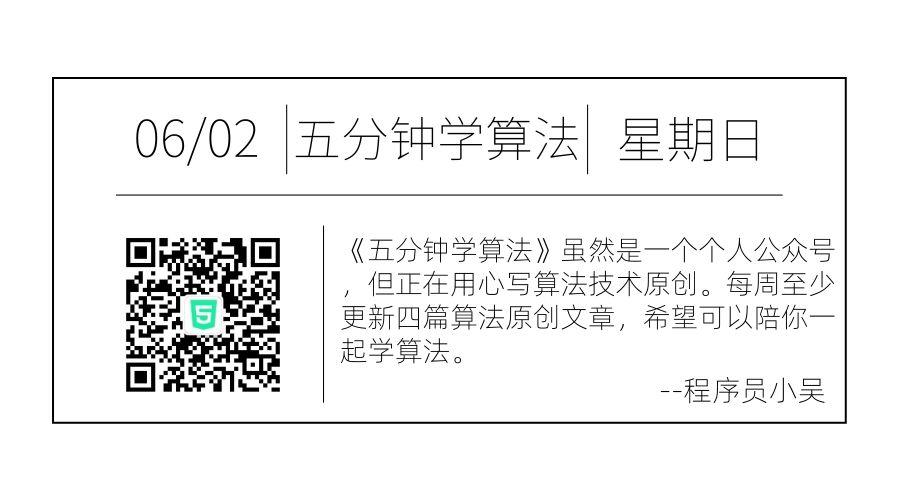5

### 2 算法流程

（1）建立数学模型来描述问题。
（2）把求解的问题分成若干个子问题。
（3）对每一子问题求解，得到子问题的局部最优解。
（4）把子问题的局部最优解合成原来问题的一个解。

### 3 伪代码

从问题的某一初始解出发
while (能朝给定总目标前进一步)
do
选择当前最优解作为可行解的一个解元素；
由所有解元素组合成问题的一个可行解。


### 题目分析

（1）建立数学模型

X1 + X2 + … + Xn = 456.
（2）问题拆分为子问题

（3）制定贪心策略，求解子问题

• 选取面值为 100 的纸币，则 X1 = 100, V = 456 – 100 = 356；
• 继续选取面值为 100 的纸币，则 X2 = 100, V = 356 – 100 = 256；
• 继续选取面值为 100 的纸币，则 X3 = 100, V = 256 – 100 = 156；
• 继续选取面值为 100 的纸币，则 X4 = 100, V = 156 – 100 = 56；
• 选取面值为 50 的纸币，则 X5 = 50, V = 56 – 50 = 6；
• 选取面值为 5 的纸币，则 X6 = 5, V = 6 – 5 = 1；
• 选取面值为 1 的纸币，则 X7 = 1, V = 1 – 1 = 0；求解结束

（4）将所有解元素合并为原问题的解

### 代码实现

const int N = 5;
int Count[N] = {5,2,2,3,5};//每一张纸币的数量
int Value[N] = {1,5,10,50,100};
int solve(int money) {
int num = 0;
for(int i = N-1;i>=0;i--) {
int c = min(money/Value[i],Count[i]);//每一个所需要的张数
money = money-c*Value[i];
num += c;//总张数
}
if(money>0) num=-1;
return num;
}